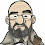## Monday, November 19, 2012

### NFL Picks Contest - Results and Updated Standings

Here are the results and updated standings in the NFL Picks Contest

lightning- Houston (W 43-37) = 16 pts.
TwoMinuteWarning- New Orleans (W 38-17) = 31 pts.
Grange95-  Atlanta (W 23-19) = 14 pts.
ohcowboy12go- Dallas (W 23-20) = 13 pts.
M Prosk- Denver (W 30-23) = 17 pts.
Full House- Houston (W 43-37) = 16 pts.
Music City Momma- Denver (W 30-23) = 17 pts.
Coach-  Houston (W 43-37) = 16 pts.
Neophyte- Cincinnati (W 28-6) = 32 pts.
Waffles- Atlanta (W 23-19) = 14 pts..
MOJO-  Houston (W 43-37) = 16 pts.
ggrouchie- Houston (W 43-37) = 16 pts.
Vegas Vic- Houston (W 43-37) = 16 pts.
Captain Crunch- New Orleans (W 38-17) = 31 pts.

Here are the current standings;

MOJO - 40+19+22+13+16= 110 pts
grrouchie - 14+48+20+5+16= 103
TwoMinuteWarning - 13+19+22+16+31= 101
Coach - 20+19+22+13+16= 90
Captain Crunch - 13+11+18+16+31= 89
Grange95 - 20+19+22+13+14= 88
Vegas Vic - 16+19+22+13+16= 86
lightning - 13+19+24+13+16= 85
Music City Momma - 17+19+24+5+17= 82
Neophyte - 17+19+0+13+32= 81
M Prosk - 17+0+41+5+17= 80
Waffles - 15+11+22+16+14= 78
ohcowboy12go - 13+19+22+5+13= 72
Full House - 20+19+22-(34)+16= 43

Congratulations to Neo for the high score this week!

1.1.